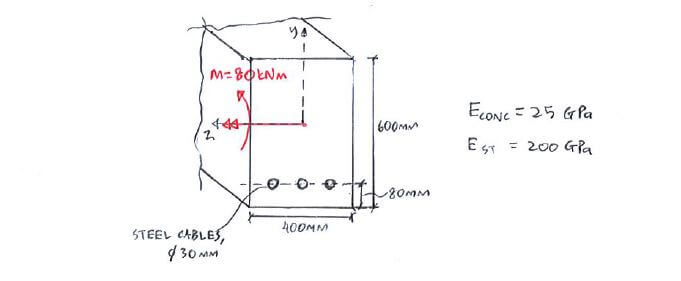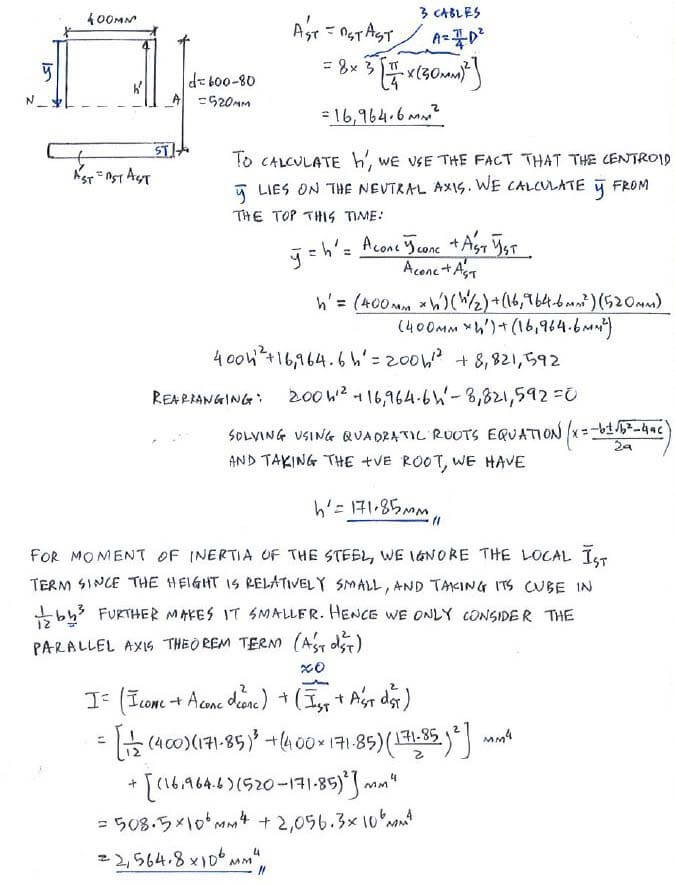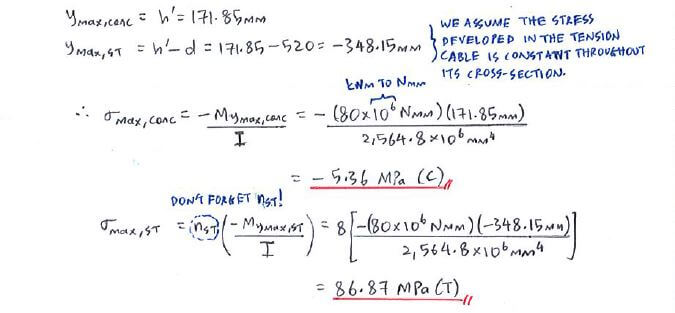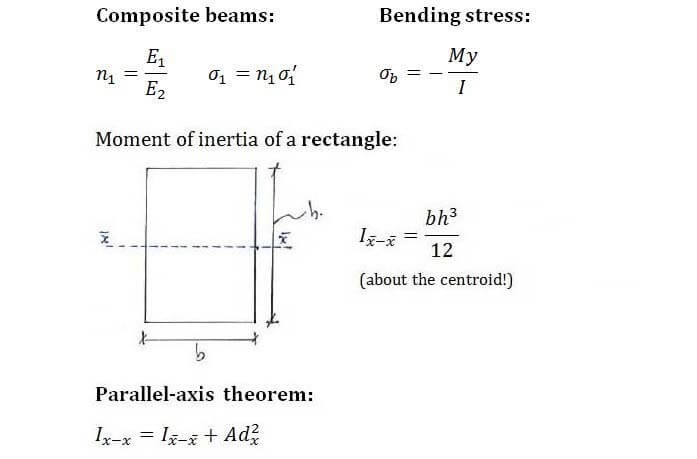# Example QuestionClick below to show answer. X

#### Step 2: Calculate section properties (h', I) Step 2: Calculate section properties (h', I)#### Step 3: Calculate σb,max for concrete and steel Step 3: Calculate σb,max for concrete and steel#### Useful formula: Useful formula:Now that you've mastered this question, you can try Question 1.